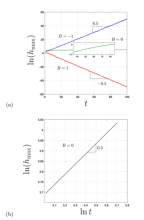On the stability of waves in classically neutral flows
By Colin Huber, Meaghan Hoitt, Nicole Hill, Kimberlee Keithley, Steven J. Weinstein, Nathaniel S. Barlow
(Wednesday, July 10, 2019)

## Abstract

This paper reports a breakdown in linear stability theory under conditions of neutral stability that is deduced by an examination of exponential modes of the form h≈ei(kx−ωt), where h is a response to a disturbance, k is a real wavenumber, and ω(k) is a wavelength-dependent complex frequency. In a previous paper, King et al (Stability of algebraically unstable dispersive flows, \textit{Phys. Rev. Fluids}, 1(073604), 2016) demonstrates that when Im[ω(k)]=0 for all k, it is possible for a system response to grow or damp algebraically as h≈ts where s is a fractional power. The growth is deduced through an asymptotic analysis of the Fourier integral that inherently invokes the superposition of an infinite number of modes. In this paper, the more typical case associated with the transition from stability to instability is examined in which Im[ω(k)]=0 for a single mode (i.e., for one value of k) at neutral stability. Two partial differential equation systems are examined, one that has been constructed to elucidate key features of the stability threshold, and a second that models the well-studied problem of rectilinear Newtonian flow down an inclined plane. In both cases, algebraic growth/decay is deduced at the neutral stability boundary, and the propagation features of the responses are examined.

## CCRG Authors

Barlow, Nathaniel S.Weinstein, Steven J.

## Research Areas

Analytic Relativity & Modeling